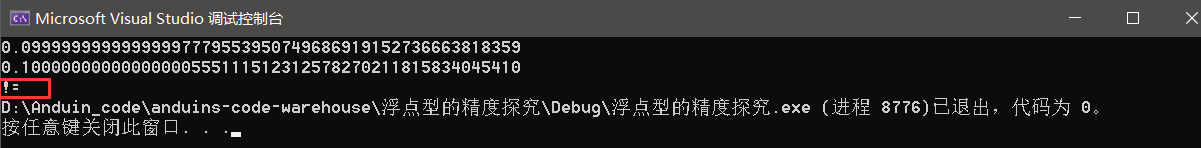0

# 【C语言】探索数据的存储(浮点型篇)

## 2. 浮点型在内存中的存储

``````3.141E10//1.0 * (10 ^ 10)
``````

``````float.h
``````## 3. 例题引入

``````intmain(){int n =9;float* pFloat =(float*)&n;printf("n的值为：%d\n", n);//9printf("*pFloat的值为：%f\n",*pFloat);//0.000000*pFloat =9.0;printf("num的值为：%d\n", n);//1091567616printf("*pFloat的值为：%f\n",*pFloat);//9.000000return0;}
``````## 4. 浮点数存储规则

• (-1)^S * M * 2^E
• (-1)^S表示符号位，当S=0，V为正数；当S=1，V为负数。
• M表示有效数字，大于等于1，小于2。
• 2^E表示指数位。

## 4.1 浮点数的存

``````5.5

101.1表示一个整数，所以S =0；

S   M     E
``````

``````S E M
``````

32位浮点数：64位浮点数：IEEE 754的特殊规定：

IEEE 754规定，在计算机内部保存M时，默认这个数的第一位总是1，因此可以被舍去，只保存后面的xxxxxx部分。

## 4.2 浮点数的取

E不全为0或不全为1：

0.5（1/2）的二进制形式为

``````0.1
``````

，由于规定正数部分必须为1，即将小数点右移1位，则为

``````1.0*2^(-1)
``````

，在内存中为-1+127=126，表示为01111110，而尾数1.0去掉整数部分为0，补齐0到23位00000000000000000000000，

E全为0：

0.000000001 * 2 ^(-126)，这是不是一个极小的数字，几乎接近于0(正负取决于符号位s)。

E全为1：

## 5. 例题解答

``````intmain(){int n =9;//补码：0000 0000 0000 0000 0000 0000 0000 1001float* pFloat =(float*)&n;//浮点型存储：0 00000000 00000000000000000001001//E为全零，E = 1 - 127 = -126//M不再加上1，M = 0.00000000000000000001001//正数，S = 0//(-1)^0 * 0.00000000000000000001001 * 2^(-126)//几乎为0printf("n的值为：%d\n", n);//存储为整形，取出来也是按照整形的方式取：9printf("*pFloat的值为：%f\n",*pFloat);//0.000000*pFloat =9.0;//二进制表示：1001.0//小数点右移三位：1.001 * 2 ^ 3//S = 0//M = 1.001//E = 3(-1)^0 * 1.001 * 2^3//浮点型存储：0 01000010 00100000000000000000000printf("num的值为：%d\n", n);//1091567616//认为内存中放的是有符号整数，符号位为0，正数//认为二进制序列就是它的原码printf("*pFloat的值为：%f\n",*pFloat);//存储为浮点型，取出来也是按照浮点型的方式取：9.000000return0;}
``````

## 6. 浮点型的精度探究(※)

(由于浮点数默认是double类型，使用float可能会出现告警，考虑到方便起见和double精度更高，所以以下内容均使用double类型)

## 6.1 浮点数的精度丢失

``````intmain(){double x =3.6;printf("%.50lf\n", x);return0;}
``````## 6.2 浮点数之间如何比较

``````intmain(){double x =1.0;double y =0.1;printf("%.50lf\n", x -0.9);printf("%.50lf\n", y);if((x -0.9)==0.1){printf("==");}else{printf("!=");}return0;}
````````````==
``````

C语言中浮点数应该进行范围精度比较！！！

``````-EPS ~ EPS
````````````fabs((x - y) < EPSLION)
``````

。fabs是一个库函数，计算变量的绝对值，相关头文件为

``````#include<math.h>
````````````#defineEPS0.00000000000001//自定义的精度，名字，数值可以自定义
``````

``````#defineDBL_EPSILON//系统提供的精度，需要引头文件#include<float.h>
````````````DBL_EPSILON
``````

``````//#define EPS 0.00000000000001//自定义方案#defineDBL_EPSILON//系统提供的精度，是一个非常小的值#include<stdio.h>#include<float.h>#include<math.h>intmain(){double x =1.0;double y =0.1;printf("%.50lf\n", x -0.9);printf("%.50lf\n", y);//if (fabs((x - 0.9) - y) < EPS)//自定义if(fabs((x -0.9)- y)< DBL_EPSILON)//系统{printf("==\n");}else{printf("!=\n");}return0;}
``````## 6.3 浮点数和0比较

``````x - y
``````

``````x - 0
``````

，直接写做x即可。

``````#include<float.h>#include<stdio.h>#include<math.h>intmain(){double x =0.0;if(fabs(x)< DBL_EPSILON)//fabs(x - 0)//if(x > -DBL_EPSILON && x < DBL_EPSILON){printf("yes\n");//一个浮点数和0比较，只要保证它的绝对值在精度范围内就相等}return0;}
````````````x >= -DBL_EPSILON && x <= DBL_EPSILON)
``````

(

``````fab(x) <= DBL_EPSILON
``````

)？

double y + x != y;也就是加上x(精度)会引起变化，相当于double y + DBL_EPSILON ！= y;

## 7. 结语### “【C语言】探索数据的存储(浮点型篇)”的评论:

##### 关于作者##### overfit同步小助手